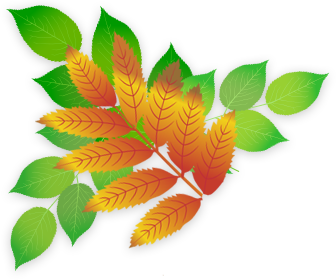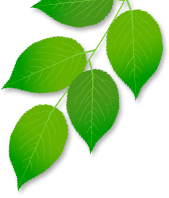## Developing Numeracy in Year 6

In Year 6 children learn to apply their mathematical skills to problem solving in a range of contexts. They work with numbers up to 10,000,000 and down to three decimal places. They learn to perform calculations using formal methods of long and short multiplication and division. They are taught how to express missing numbers in algebraic problems and use simple formulae to express number sequences and solve problems. They are shown how to generate and use formulae to calculate the area and volume of shapes. They will know properties of circles and the relationships between diameter, radius and circumference. Children are taught how to calculate percentages of quantities and relate percentages to decimals and fractions.

These are the objectives that children will be taught in Numeracy during Year 6.

Year 6: Number and Place Value

•  read, write, order and compare numbers up to 10 000 000 and determine the value of each digit
•  round any whole number to a required degree of accuracy
•  use negative numbers in context, and calculate intervals across zero
•  solve number problems and practical problems that involve all of the above.

• multiply multi-digit numbers up to 4 digits by a two-digit whole number using the formal written method of long multiplication
• divide numbers up to 4 digits by a two-digit whole number using the formal written method of long division, and interpret remainders as whole number remainders, fractions, or by rounding, as appropriate for the context
• divide numbers up to 4 digits by a two-digit number using the formal written method of short division where appropriate, interpreting remainders according to the context
• perform mental calculations, including with mixed operations and large numbers
• identify common factors, common multiples and prime numbers
• use their knowledge of the order of operations to carry out calculations involving the four operations
• solve addition and subtraction multi-step problems in contexts, deciding which operations and methods to use and why
• solve problems involving addition, subtraction, multiplication and division
• use estimation to check answers to calculations and determine, in the context of a problem, an appropriate degree of accuracy.

Year 6: Multiplication and Division

• multiply multi-digit numbers up to 4 digits by a two-digit whole number using the formal written method of long multiplication
• divide numbers up to 4 digits by a two-digit whole number using the formal written method of long division, and interpret remainders as whole number remainders, fractions, or by rounding, as appropriate for the context
• divide numbers up to 4 digits by a two-digit number using the formal written method of short division where appropriate, interpreting remainders according to the context
• perform mental calculations, including with mixed operations and large numbers
• identify common factors, common multiples and prime numbers
• use their knowledge of the order of operations to carry out calculations involving the four operations
• solve addition and subtraction multi-step problems in contexts, deciding which operations and methods to use and why
• solve problems involving addition, subtraction, multiplication and division
• use estimation to check answers to calculations and determine, in the context of a problem, an appropriate degree of accuracy.

Year 6: Fractions (including decimals and percentages)

• use common factors to simplify fractions; use common multiples to express fractions in the same denomination
• compare and order fractions, including fractions >1
• add and subtract fractions with different denominators and mixed numbers, using the concept of equivalent fractions
• multiply simple pairs of proper fractions, writing the answer in its simplest form [for example, ¼ × ½ = 1⁄8]
• divide proper fractions by whole numbers [for example, 1⁄3 ÷ 2 = 1⁄6]
• associate a fraction with division and calculate decimal fraction equivalents [for example, 0.375] for a simple fraction [for example, 3⁄8]
• identify the value of each digit in numbers given to three decimal places and multiply and divide numbers by 10, 100 and 1000 giving answers up to three decimal places
• multiply one-digit numbers with up to two decimal places by whole numbers
• use written division methods in cases where the answer has up to two decimal places.
• solve problems which require answers to be rounded to specified degrees of accuracy
• recall and use equivalences between simple fractions, decimals and percentages including in different contexts.

Year 6: Ratio and Proportion

• solve problems involving the relative sizes of two quantities where missing values can be found by using integer multiplication and division facts
• solve problems involving the calculation of percentages [for example, of measures, and such as 15% of 360] and use percentages for comparison
• solve problems involving similar shapes where the scale factor is known or can be found
• solve problems involving unequal sharing and grouping using knowledge of fractions and multiples

Year 6: Measurement

• solve problems involving the calculation and conversion of units of measure, using decimal notation up to three decimal places where appropriate
• use, read, write and convert between standard units, converting measurements of length, mass, volume and time from a smaller unit of measure to a larger unit, and vice versa, using decimal notation to up to three decimal places
• convert between miles and kilometres
• recognise that shapes with the same areas can have different perimeters and vice versa
• recognise when it is possible to use the formulae for area and volume of shapes
• calculate the area of parallelograms and triangles
• calculate, estimate and compare volume of cubes and cuboids using standard units, including cubic centimetres (cm3) and cubic metres (m3), and extending to other units [for example, mm3 and km3]

Year 6: Geometry - properties of shapes

• draw 2-D shapes using given dimensions and angles
• recognise, describe and build simple 3-D shapes including making nets
• compare and classify geometric shapes based on their properties and sizes and find unknown angles in any triangles, quadrilaterals, and regular polygons
• illustrate and name parts of circle, including radius, diameter and circumference and know that the diameter is twice the radius
• recognise angles where they meet at a point, are on a straight line, or are vertically opposite, and find missing angles.

Year 6: Geometry - position and direction

• describe positions on the full coordinate grid (all four quadrants)
• draw and translate simple shapes on the coordinate plane, and reflect them in the axes

Year 6: Statistics

• interpret and construct pie charts and line graphs and use these to solve problems
• calculate and interpret the mean as an average

Year 6: Algebra

• use simple formulae
• generate and describe linear number sequences
• express missing number problems algebraically.
• find pairs of numbers that satisfy number sentences involving two unknowns
• enumerate possibilities of combinations of two variables